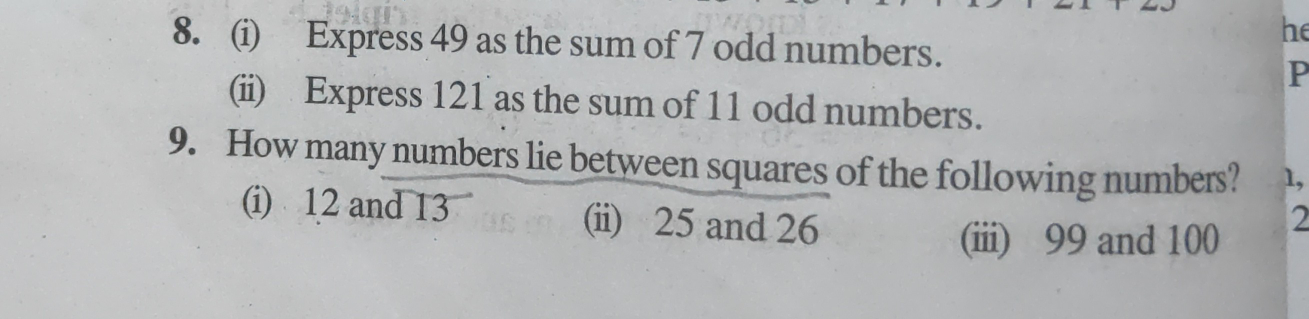# 8.(i) Express 49 as the sum of 7 odd numbers.(ii) Express 121 as the sum of 11 odd numbers.9. How many numbers lie between squares of the following numbers?(i) 12 and 13(ii) 25 and 26(iii) 99 and 100"

8. (i) Given:  Express 49 as the sum of 7 odd numbers.

To find: We have to express 49 as the sum of 7 odd numbers

Solution:

1 + 3 + 5 + 7 + 9 + 11 + 13 = 49

8. (ii)

Given:   Express 121 as the sum of 11 odd numbers.

To find: We have to express  121 as the sum of 11 odd numbers

Solution:1 + 3 + 5 + 7 + 9 + 11 + 13 + 15 + 17 + 19 + 21 = 121

9.(i)

Given: 12 and 13.

To find: We have to find how many numbers lie between squares of 12 and 13.

Solution:

122 = 144

132 = 169

Now,

169 - 144 = 25

So, the number between squares of 12 and 13 is 25 - 1 = 24

9. (ii)

Given:  25 and 26

To find: We have to find how many numbers lie between squares of 25 and 26.

Solution:

252 = 625

262 = 676

Now,

676 - 625 = 51

So, number between squares of 25 and 26 is 51 - 1 = 50

9. (iii)

Given: 99 and 100.

To find: We have to find how many numbers lie between squares of 99 and 100.

Solution: 99 and 100

992 = 9801

1002 = 10000

Now,

10000 - 9801 = 199

So, number between squares of 99 and 100 is 199 - 1 = 198.